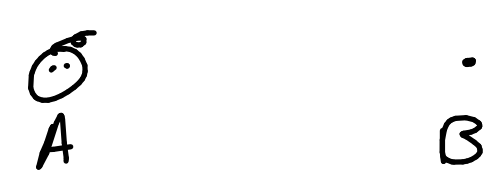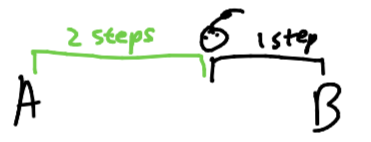# Chapter 1: Baby Steps

As promised, this tutorial on recursion will start with the basics. It's still a new topic, so don't be discouraged if you struggle.

### Recursion problem #1: Step

Imagine a baby that's at point A, and the baby wants to get to point B.Let's say the baby is around... 3 steps away from point B. For the sake of simplicity,
Point B = Point A + 3 stepsSeems manageable so far, right? Let's try to frame this problem in a recursive mindset... and to do that, we'll define a function step which aims to take the baby from point A to point B. The function has one parameter, baby_location, which is the baby's current location. (This isn't going to be working code, just a framework for us to think inside of.)
def step(baby_location):
#this doesn't do anything...
In computer science, recursion refers to a function that uses itself inside its own definition. So, if we're solving this problem recursively, we're going to have to call step inside the definition somewhere. This might seem weird, but lets see what that looks like.
def step(baby_location):
step(baby_location)
What happens when we call step(point_A)?
step would call itself endlessly; that doesn't seem good! The baby would just stay at point A like so...
step(point_A) -> step(point_A) -> step(point_A) -> step(point_A) -> step(point_A) -> ...
However, if you think about it, a function that repeats over and over again is actually really useful for solving problems.
For example, you've seen this before in the form of while loops:
#increase x from 0 to 3
x = 0
while x != 3:
x = x + 1
This loop repeatedly executes `x = x + 1` until x is 3. What's important about this is that:
1. 1.
every time it loops, it gets x closer to 3
2. 2.
it stops when x = 3
Going back to step, step could be useful to us if it had the same properties:
1. 1.
Each time we step, the baby gets closer to point B
2. 2.
The program stops when the baby has reached point B
Let's try it out.
As a reminder, this is our baby:If we want our baby to get closer to point B, we should probably tell it to take a step closer to point B!Wow! Now our baby is only 2 steps away from point B! We've successfully allowed the baby to get closer to point B.
Let's represent that in our code:
def step(baby_location):
step(baby_location + 1_step)
As a reminder, this code makes no sense in real life, but the idea should be clear. Every time we step, we calculate the baby's location if it was one step closer, and then we step again with the new location. The parameter, baby_location, comes closer to Point B, step by step.
step(point_A) -> step(point_A + 1_step) -> step(point_A + 1_step + 1_step) -> ...
Let's continue stepping!almost there...But with our current code, the baby would keep stepping past point B. He's just a baby, he doesn't know when to stop! So, let's tell him when to stop.
def step(baby_location):
if baby_location == point_B:
return
step(baby_location + 1_step)
Now, we check first if the baby has reached point B, at which point the function should stop, so we return. This time, we don't reach the call to step again, so the program ends. And we're done!
If you're still a bit confused, here's a recap to help you understand what's happening:
step(point_A) -> Is baby at point B? NO -> step(point_A + 1_step)
step(point_A + 1_step) -> Is baby at point B? NO -> step((point_A + 1_step) + 1_step) -> step(point_A + 2_step)
step(point_A + 2_step) -> Is baby at point B? NO -> step((point_A + 2_step) + 1_step) -> step(point_A + 3_step)
step(point_A + 3_step) -> Is baby at point B? YES -> return

### How is this useful?

You might be thinking to yourself, "Well, sure. I've written a recursive function that moves a baby from point A to point B. How is that ever going to help me?" The answer to that is that the basic ideas behind this function are the exact same for nearly every recursive function you will write.
As a reminder, the properties of step that allowed us to solve the problem were:
1. 1.
Each time we call step, the baby gets a little closer to point B
2. 2.
The program ends when the baby reaches point B
In fact, if we generalize these two properties, we can use them as a guideline for solving all problems recursively:
1. 1.
Each time we call our recursive function, we make the problem smaller.
2. 2.
When the problem is solved or is small enough to be solvable instantly, we finish the problem and stop.
In most kinds of recursion you'll encounter, we make the problem smaller inside the recursive call. And when we check if the problem is solved or solvable instantly, we are considering whether any of the base cases have been reached. If you can identify these two things, you are well on your way to mastering recursion.